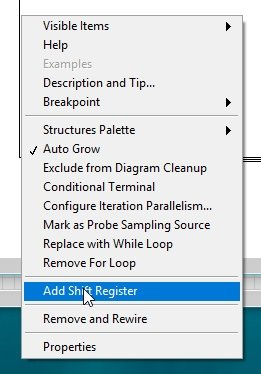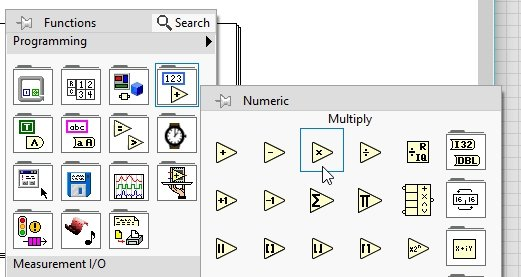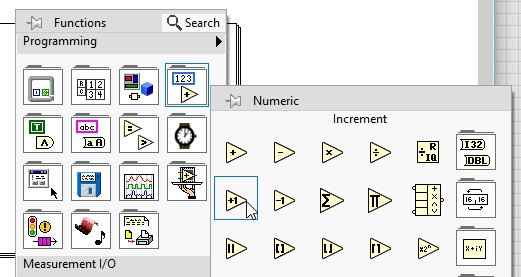# Calculate Factorial of a number in LabView : tutorial 35

In this tutorial I will help you to design a VI that will take a number as input from the user and at the output it will return the factorial of that natural number. For example, the user enters 3 as an input value to the VI then the output of the VI will return 1*2*3 = 6 in the indicator. We will be using a ‘for’ loop to do so. At the end of the tutorial you are provided with an exercise to do it by yourself.

### Calculate Factorial of a number in LabView

Figure 1: Input control

Figure 2: For loop placement

Figure 3: Number of loops

• We can also use while loop as we used in the previous tutorial of sum calculation, its’ up to us which loop we prefer.
• If we are willing to multiply all previous number of a numeric integer which is the demand of factorial calculations we will need the previous product value of the loop. But how can we access the previous value of the product??. We can do so by using shift registers as we have used in previous tutorial. Click right on the boundary of the for loop and from the drop down menu select ad shift register as shown in the figure below,Figure 5: Numeric constant placement

Figure 6: Shift register constant

• You might be wondering that we used 0 as the initial value of shift register in case of addition but now we are using 1, what is the difference. If we use 0 here as the initial condition then the shift register will multiply the 0 with all the previous products and the result of factorial will be 0.
• Do overcome this ambiguity we use 1 as an initial condition. For the multiplication task place a multiply block, from the function palette select numeric and then select multiply as shown in the figure below,Figure 7: Placement of multiply block

• At one of the two inputs of the multiply block connect the right shift register, and the output of this block connect the right shift register. The purpose of this will be, the right shift register will store the value of product from the previous iteration and will pass it on to the left shift register in the next iteration, as we have discussed in detail in the tutorial related to shift registers.
• Now on the other input of the multiply block we have to connect the incremented iteration because the number of iterations start from 0 but we want the factorial from 1 onwards, because the factorial will turn out to be zero otherwise From the function palette select numeric and then select increment as shown in the figure below,Figure 8: Increment block placement

Figure 9: Factorial calculation block diagram

Figure 10: Output indicator placement

Figure 11: Complete block diagram

Figure 12: Output 1

Figure 13: Output 2

Exercise:

• Design a VI that will take two input integers and calculate the Product of natural numbers between those two integers

<< Previous tutorial                                                  Next tutorial>>